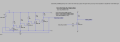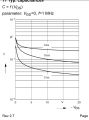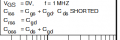# Calculating gate delay PMOS ring oscillator

Thread Starter

#### daanmicro

Joined Nov 15, 2017
50
Hi there,

I've developed a ring oscillator (sort of a school project):I used the following circuit for this:MOSFET: https://assets.nexperia.com/documents/data-sheet/BSS84.pdf

Now when is connect this SMD circuit to an oscilloscope, i've got a frequency of 260 kHz. In the simulation in spice i've got a frequency of 187 kHz. That's fine.

Now when i want to calculate the frequency by myself:
Ring Oscillator: 1/(2*n*t(p)) ___ n = amount of gates,,, t(p) is gate delay
i get really weird numbers for the frequency or gate delay.
e.g. when i fill in the frequency in this formula, for 5 gates. I get a gate delay of around 40 us, which in my idea is way too much.

I've also found another formula: t(p) = (C*dV)/I(d)
still i get really weird numbers out of these formulas. Can anybody help me out with this?

Thanks in advance! I hope i've been clear enough.

Daan

#### Bordodynov

Joined May 20, 2015
2,867
F~k/(5*(0.04n*2+0.02n)*10k), k~1 ==> 200kHz

Thread Starter

#### daanmicro

Joined Nov 15, 2017
50
So i'm assuming you take 5 * tau (or a sort of timeconstant, just R*C), but why 2*0.04n instead of just 0.04n. Im not very familiar with electronics.

Thanks again

#### Bordodynov

Joined May 20, 2015
2,867
I am sorry.
Tau=k*(a*Cgd_avg+Cgs+Cds_avg)
Study the effect of Miller. At the input of the transistor and the output of the transistor, there are equal in magnitude pulses. Those. we can approximately assume that the gain is -1 (with a small phase shift). Therefore, the capacity is approximately doubled.
cgdmin<Cgd_avg<cgdmax, Cds_avg<Cjo.

Thread Starter

#### daanmicro

Joined Nov 15, 2017
50
Great, thanks a lot (ofcourse there's no need to be sorry!). Now i understand the calculation from an LTspice simulation. For my report i also wanted to do a theoretical approach from the datasheet of the transistor. When i look at the 'typical capacitance' graph of the transistor i've used:These capacitances are given, but I don't really understand how to convert these capacitances the right way into the formula. I've found the following in another datasheet:Not sure if this always applies. But still i can't come to a corresponding result of a theoretical approach.

You would really fix my grade by helping me figure this out. I've tried some books about it, but you don't see a lot of PMOS oscillators, mostly CMOS without a resistor. I understand it's not possible to exactly know the capacitance, but with the Vds and the typical capacitance graph from the datasheet, i thought it would be possible to do a representative estimation of the frequency (or so gate delay).

Thanks a lot lot lot!

#### crutschow

Joined Mar 14, 2008
27,194
What you really need to use for digital circuits to determine the frequency, is the total gate charge that needs to be transferred, since capacitance varies with gate voltage.
From that you can calculate approximately how long it takes the drain resistor to charge the gate to the gate threshold voltage (the switching point).
The gate discharge is much faster since that is determined by the drain-source ON resistance.
If you look at the simulation, you will see this.Last edited:

#### Bordodynov

Joined May 20, 2015
2,867

#### Attachments

• 3.2 KB Views: 3